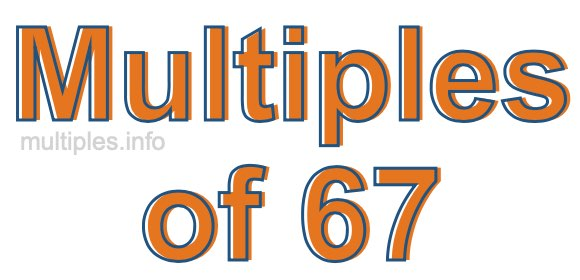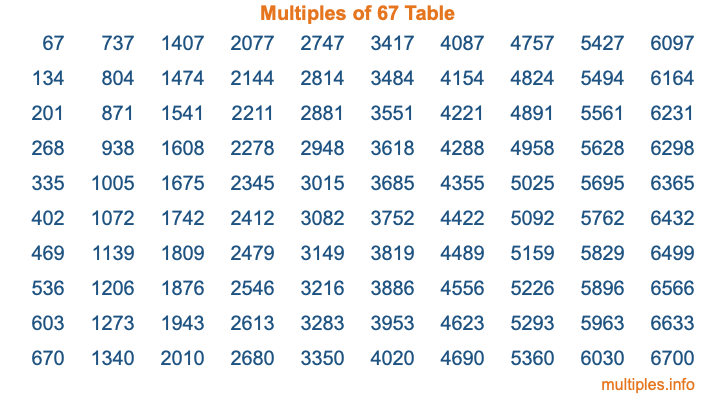Multiples of 67Welcome to the Multiples of 67 page. Here we will first teach you everything you will ever need to know about the multiples of 67, and then give you a study guide summary of everything we taught you to make sure you remember it all. Use this page to look up facts and learn information about the multiples of 67. This page will make you a multiples of sixty-seven expert!

Definition of Multiples of 67
Multiples of 67 are all the numbers that when divided by 67 equal an integer. Each of the multiples of 67 are called a multiple. A multiple of 67 is created by multiplying 67 by an integer.

Therefore, to create a list of multiples of 67, you start with 1 multiplied by 67, then 2 multiplied by 67, then 3 multiplied by 67, and so on for as long as you want. Thus, the list of the first five multiples of 67 is 67, 134, 201, 268, and 335. To see a larger list of multiples of 67, see the printable image of Multiples of 67 further down on this page. We also have a category where you can choose any nth multiple of 67.

Multiples of 67 Checker
The Multiples of 67 Checker below checks to see if any number of your choice is a multiple of 67. In other words, it checks to see if there is any number (integer) that when multiplied by 67 will equal your number. To do that, we divide your number by 67. If the the quotient is an integer, then your number is a multiple of 67.

Is  a multiple of 67?

Least Common Multiple of 67 and ...
A Least Common Multiple (LCM) is the lowest multiple that two or more numbers have in common. This is also called the smallest common multiple or lowest common multiple and is useful to know when you are adding our subtracting fractions. Enter one or more numbers below (67 is already entered) to find the LCM.

Check out our LCM Calculator if you need more details about the Least Common Multiple or if you need the LCM for different numbers for adding and subtraction fractions.

nth Multiple of 67
As we stated above, 67 is the first multiple of 67, 134 is the second multiple of 67, 201 is the third multiple of 67, and so on. Enter a number below to find the nth multiple of 67.

th multiple of 67

Multiples of 67 vs Factors of 67
67 is a multiple of 67 and a factor of 67, but that is where the similarities end. All postive multiples of 67 are 67 or greater than 67. All positive factors of 67 are 67 or less than 67.

Below is the beginning list of multiples of 67 and the factors of 67 so you can compare:

Multiples of 67: 67, 134, 201, 268, 335, etc.

Factors of 67: 1, 67

As you can see, the multiples of 67 are all the numbers that you can divide by 67 to get a whole number. The factors of 67, on the other hand, are all the whole numbers that you can multiply by another whole number to get 67.

It's also interesting to note that if a number (x) is a factor of 67, then 67 will also be a multiple of that number (x).

Multiples of 67 vs Divisors of 67
The divisors of 67 are all the integers that 67 can be divided by evenly. Below is a list of the divisors of 67.

Divisors of 67: 1, 67

The interesting thing to note here is that if you take any multiple of 67 and divide it by a divisor of 67, you will see that the quotient is an integer.

Multiples of 67 Table
Below is an image of the first 100 multiples of 67 in a table. The table is in chronological order, column by column. The first column has the first ten multiples of 67, the second column has the next ten multiples of 67, and so on.The Multiples of 67 Table is also referred to as the 67 Times Table or Times Table of 67. You are welcome to print out our table for your studies.

Negative Multiples of 67
Although not often discussed or needed in math, it is worth mentioning that you can make a list of negative multiples of 67 by multiplying 67 by -1, then by -2, then by -3, and so on, to get the following list of negative multiples of 67:

-67, -134, -201, -268, -335, etc.

Multiples of 67 Summary
Below is a summary of important Multiples of 67 facts that we have discussed on this page. To retain the knowledge on this page, we recommend that you read through the summary and explain to yourself or a study partner why they hold true.

There are an infinite number of multiples of 67.

A multiple of 67 divided by 67 will equal a whole number.

67 divided by a factor of 67 equals a divisor of 67.

The nth multiple of 67 is n times 67.

The largest factor of 67 is equal to the first positive multiple of 67.

67 is a multiple of every factor of 67.

67 is a multiple of 67.

A multiple of 67 divided by a divisor of 67 equals an integer.

67 divided by a divisor of 67 equals a factor of 67.

Any integer times 67 will equal a multiple of 67.

Multiples of a Number
Here you can get the multiples of another number, all with the same attention to detail as we did for multiples of 67 on this page.

Multiples of
Multiples of 68
Did you find our page about multiples of sixty-seven educational? Do you want more knowledge? Check out the multiples of the next number on our list!